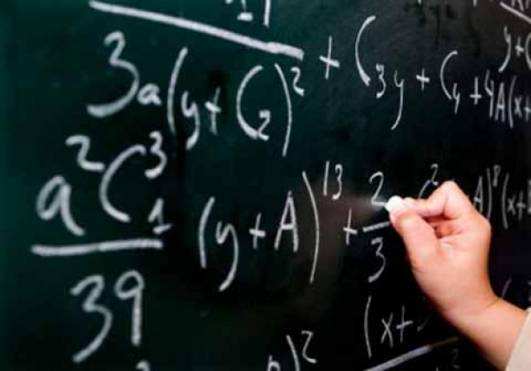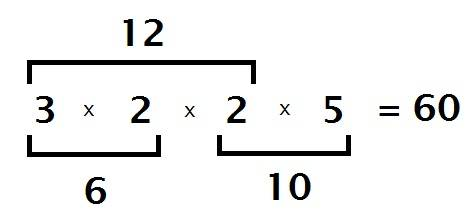What is the lcm of 12,6,and 10?

Finding the LCM (Lowest Common Multiple) of any set of numbers requires you to first find the factors.The factors are the whole numbers you can multiply together to get a particular number. For example, if you multiply 2 and 3 you get 6. So 2 and 3 are FACTORS of 6. However its possible that there may be two factors that are the same number. For 12, the factors are (2, 2, 3). Two is in their twice because if you left out a 2 you would get 6 not twelve when the numbers were multiplied together. The factors are as follows:

6 = 2 x 3

12 = 2 x 2 x 3

10 = 2 x 5

The LCM is when you include all of the factors above. So you would take 3 and 5. Since 12 has multiple 2's, your LCM needs to as well. So the resulting set of numbers is (2, 2, 3, 5). The order of the numbers doesn't matter. If you multiple the numbers together you get the LCM:So for 6, 10 and 12 the LCM is 60. You can take any of the three numbers and they will divide evenly into the LCM. This is how you can test to make sure you got the right answer. If we had only included a single 2 then we would have multiplied 2 x 3 x 5 = 30. When we try to divide 30 by 12 we don't receive a whole number. This tells us the answer is wrong.

30 / 12 = 2.5

60 / 12 = 5

thanked the writer.